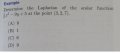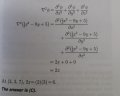# Studying for FE: Help Understanding Laplacian Math Question

#### al seeger

Joined Dec 9, 2014
13
Hello Everyone.
I am currently in the process of studying for the FE (Electrical and Comp) Exam.
Trying to learn some concepts I missed in school and was hoping for some advice with the following question:The Solution is as follows:

So far I've done little more than copy the problem. I'm just not sure where to go from there, if someone could help me understand the solution, I'd be grateful. Thanks and Best Regards.
- Al -#### WBahn

Joined Mar 31, 2012
29,489
What part of the given solution is stumping you? Do you know how to take partial derivatives?

#### al seeger

Joined Dec 9, 2014
13
Thank you WBahn for the quick reply.
No, I don't believe I do. However, my next step will be to learn the partial derivative procedure, if that's what you suggest.
I'll post back later after trying that.
Best Regards.

#### WBahn

Joined Mar 31, 2012
29,489
What background in calculus do you presently have?

#### al seeger

Joined Dec 9, 2014
13
I took Cal II a few years ago. I do remember briefly going over this subject.... guess it didn't stick .

#### WBahn

Joined Mar 31, 2012
29,489
Where's the x² in the denominator coming from?

#### al seeger

Joined Dec 9, 2014
13
Correction: I meant to say "Partial Derivatives" not "Integration by parts"

It looks to me as the denominator (x^2) is used to evaluate each part of the equation at x, y, and z

Apparently (a*f)' = a*f' ==> 1/3 a/ax x^3

Then apply the Power Rule: 1/3 3x^3-1 ==> x^2
?

#### Papabravo

Joined Feb 24, 2006
20,595
No. The differential operator

$$\frac{\partial ^2}{\partial x^2}$$ means: take the partial derivative with respect to x, and x only, twice. There is no $$x^2$$ in the denominator.

#### WBahn

Joined Mar 31, 2012
29,489
I'm not seeing it.

You want the second partial derivative with respect to x of f(x). So take the partial derivative with respect to x twice.

$$f(x) \; = \; \frac{1}{3}x^3 \, - \, 9y \, + \, 5$$

So you want

$$\frac{\partial \, f(x)}{\partial x} \; = \; \frac{\partial \,\frac{1}{3}x^3 \, - \, 9y \, + \, 5}{\partial x}$$

$$\frac{\partial \, f(x)}{\partial x} \; = \; \frac{1}{3}\frac{\partial}{\partial x} x^3 \, - \, 9y \frac{\partial}{\partial x} 1 \, + \, 5 \frac{\partial}{\partial x} 1$$

The last two terms do not depend on x at all, and are therefore zero. The first term reduces

$$\frac{\partial \, f(x)}{\partial x} \; = \; \frac{1}{3}3x^2 \; = \; x^2$$

Now take the partial derivative of this with respect to x to get the second partial derivative of f(x) with respect to x and you get

$$\frac{\partial^2 \, f(x)}{\partial x^2} \; = \; \frac{\partial}{\partial x} x^2 \; = \; 2x$$

You still need to take the second partials with respect to the other two variables, but when you do you will see that nothing survives.